Back
Excel Practice
Addition and the SUM Function in Excel
> ... Math > Excel > Addition and the SUM Function in Excel

You can watch the video or just read the text below. Both will give you the same information.

You can use Excel as a calculator to add numbers by clicking on any cell and typing the = symbol and then the numbers you want to add together separated by a + symbol.

Example: Add $$9+7$$ using Excel.

In any cell, type “=9+7” (without the quotation marks).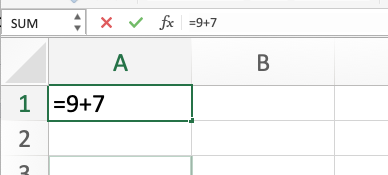When you select enter, the number 16 will appear in the cell because $$9+7=16$$.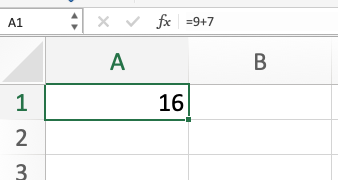Notice that the equation in the formula bar for the cell still says “=9+7”.

You can add as many numbers together as you want, similar to using a calculator, using this approach.

## Adding by Referencing Cells in the SUM Function

Another way to add numbers in Excel is to use the SUM function. To use the SUM function, type =sum() into a cell. The cell references of the cells you want to add go within the parentheses.

The AutoSUM button, which looks like the Greek letter sigma, will automatically put the SUM function into a cell.

$$\boldsymbol{\sum}$$

There are several ways to reference cells within the SUM function.

## Comma Separated Values

This is used to add the values of specific cells together. The cell references are separated by commas within the function. These cells don’t have to be next to each other in the spreadsheet.

Example: Add the values of cells A2 and A3 together.

A2 has the value 9 in it.

A3 has the value 7 in it.

Type “=sum(A2,A3)” (without quote marks) into another cell.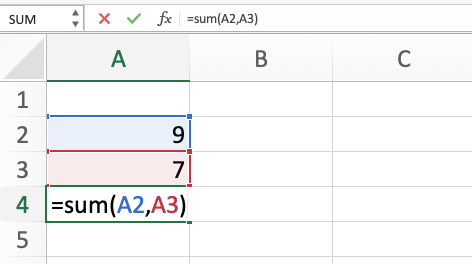Press enter to finish the formula. The value 16 will appear in the cell with the SUM function. In the formula bar you will still see the formula “=sum(A2,A3)” as the formula calculating the value of the cell. If the numbers in A2 or A3 change, the value of the sum function will change too.

## Colon Separated Values

Excel can add all the values in a vertical or horizontal group of cells using the SUM function.

To add the cells in a group, start with =sum(). With the cursor inside the parentheses of the SUM function, select the first cell and drag the cursor to the last cell in the group. This will automatically put the reference name of the first cell and the reference name of the last cell in the group, separated by a colon.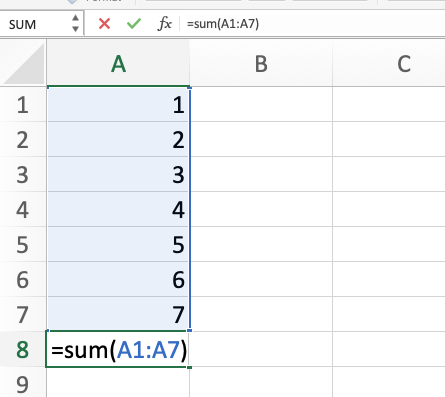Select enter to display the value of the cells added together.

The picture above adds the values in cells A1 through A7 and sums them in cell A8.

The same technique works to sum a row horizontally.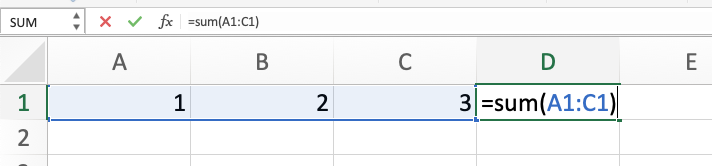## Quickly See the SUM in Bottom Right Corner

You can see the sum of several cells without actually adding them together by highlighting the cells and looking in the bottom right corner of Excel.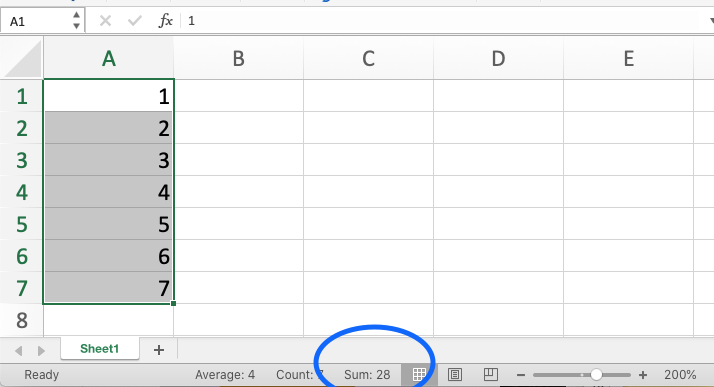## Need More Help?

1. Study other Math Lessons in the Resource Center.
2. Visit the Online Tutoring Resources in the Resource Center.